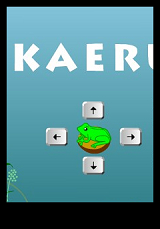Ando-san知道我最喜歡玩益智的小遊戲。

（15顆球，其中一顆比較重，如何秤3次找出比較重的球）

（後來都在玩zoo keeper，一個遊戲玩了好幾年，應該支持一下買正版遊戲片）

『如何用四個4，以加減乘除的方式，得到 5？』

===== 以下有解答 ====

1 = (4+4) / (4+4)

2 = (4*4) / (4+4) = (4/4) + (4/4) = (4/(4+4))*4

6 = 4 + ((4+4)/4)

3也還算容易：

3 = (4+4+4) / 4

5花了點時間：

5 = (4*4+4) / 4

7 = 4 + 4 - (4/4)

8 = (4/4) * (4+4)

9 = 4 + 4 + (4/4)